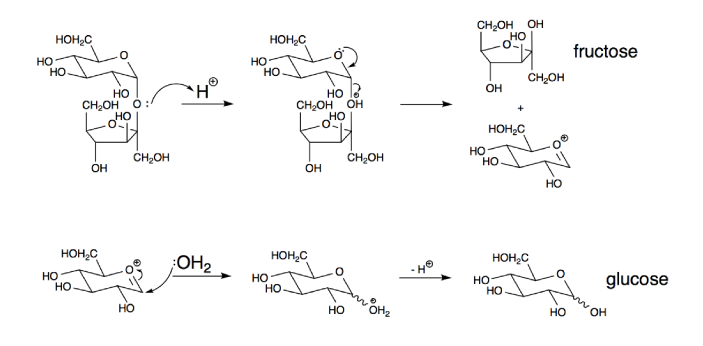# Chemistry Questions and Answers (Q&A)#### kashif

The sum of the coefficients is basically the total number of reacting molecules and products formed. However, you need to balance the equation first in order to reach the right value. For that, balance the aluminum with the oxygen and the product side.

This will give use 4 aluminum atoms reacting with 3 oxygen molecules to form 2 molecules of AL2O3. We can easily find the sum by adding all the molecules. 4+3+2=9. The sum of coefficients for this equation is 9. You can find the coefficient for other equations using this method as well. Simply balance the equation and then add the molecules on each side of the equation.#### thomasthegreat

The correct answer is answering E: the atoms have the same atomic number, but a different number of neutrons. This is key. The atomic number signifies how many protons an atom has. If it has three protons, the atomic number is three. When the electrons are greater or less than the protons, the atom has an electrical charge. If the protons and electrons are equal, then the atom has no charge.

Neutrons are special because they are not electrically charged. The only difference to anything that they can make is a difference in how heavy something is. So, one atom of uranium can have a certain amount of neurons and be radioactive, while another atom may have fewer and be completely stable.#### Eddy

Given: Sea water contains roughly 28.0 g of NaCl per liter.

To find: What is the molarity of sodium chloride (NaCl) in sea water?

Solution:

Molecular mass of NaCl= 58.4

Therefore 1 mole of NaCl= 58.4 g

Number of moles in sea water= 28/58.4= 0.479

Therefore, 1 litre of water contains 0.479 moles of NaCl

Now, Molarity(M) = Number of moles of solute/ volume of solvent.

Therefore,

M= 0.479/1 = 0.479M#### jagran josh

Option B - increase in pressure.
The extent of adsorption is directly proportional to the pressure. Therefore, increase in pressure will cause increase in rate of adsorption.

Keep in mind that temperature also has a role to play in the rate of adsorption. At low temperature, the extent of adsorption increases rapidly with pressure.#### CECHS

The volume occupied by the Ammonia gas is C. 67.2 Liters. Ammonia is a compound that is composed of both nitrogen and hydrogen. Ammonia is normally used in order to rouse people into consciousness when they faint. Take note that Ammonia gas can be dangerous when it is inhaled in large amounts. It can also be problematic when it is consumed in liquid form.

This sometimes comes in liquid form too but it should not be mixed with certain compounds in any way. For example, when this becomes exposed to bleach, expect that a type of poisonous gas can be formed and this will be deadly for a lot of people. This should be handled carefully at all times.#### j_black

In a math word problem, it is common to use a formula. In this case when the math word problem includes absorbance and wave length, then the formula should be used where the absorbance amount is equal to the amount of the molar absorptivity multiplied by the pathlength and multiplied that by the concentration amount. With that said, the problem gives the amount being absorbed, so the answer on the left of the equal side is given at 0.40. The light path is 2 centimeters and it is reduced to 1 cm.

When plugging in the numbers, the answer will come out to be 0.20 based on having to divide 0.40 by the light path amount of two after moving some of the numbers around to get the variable on one side.#### mcochran

That's weird. My answer is 3!#### mcochran

None of the choices has the correct answer to this item. The correct formula for Lead IV Nitrate is Pb(NO3)4. Pb is the chemical symbol for the element Lead and NO3 for Nitrate. If they are to form a compound it will be Pb 4+ and (NO3)-. In-laws of chemistry, the ions in the lead must be put at the end of the compound it is combined to.

That is how the answer Pb(NO3)4 forms into. And base on the given choices, there is no Pb(NO3)4 but only Pb2(NO3)2, Pb(NO3)2, which is Lead II Nitrate, and Pb4(NO3)2. Lead is soft, malleable, and is resistant to corrosion while nitrate is a salt of nitric acid.#### B. Wright

Always excited to learn and talk about new topics

Sucrose and Fructose are both known to be sweet, but they have some differences with each other. The sucrose is known to be a type of disaccharide that has one glucose and one fructose molecule. On the other hand, the sucrose is known to be the type of sugar that you will usually find in processed food.According to a lot of studies, sucrose is known to be the “bad” type of sugar, and it should be avoided at all costs. There are also some studies about Fructose because people are still trying to test if this is bad for the health, but so far, people’s diet would even need a bit of Fructose so that it can be more balanced.#### Marshall_Bailey

Different atoms have different configurations depending on the shell of electrons surrounding them. When these atoms come together in different forms to form molecules, they give rise to different molecular configurations as well. Among the different configuration, BrF4 is a molecular structure consisting of bromine and fluorine.

The molecular geometry of this molecule is square planar as this how the different lone pairs of both atoms adjust with one another. The four fluorine atoms occupy different positions around bromine giving it a planar shape while occupying four corners. Hence, the ultimate geometry is named the square planar shape of BrF4.